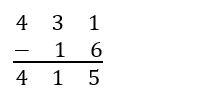# Two Digit from Three Digit Subtraction Worksheets

Tips for Two Digit from Three Digit Subtraction When you are about to teach your children about subtraction, you have to extra watchful during the subtraction of a 2-digit number for a 3-digit number. However, your students should be aware of the concept of place values beforehand. Since, it will further help them to understand the concept Since we will place the value ones and tens of the 2-digit value below of those from the 3-digit ones, let’s take an example.Here, you need to focus on the numbers you need to borrow. For instance, we needed to borrow from 3, since one is less than 6. Keep practicing, and the kids will get the hang of it.

• ### Basic Lesson

Walks students through the basic four steps of this problem type. 1. Subtract ones. 2. Subtract tens. 3. Carry down the hundreds. 4. Complete.

• ### Intermediate Lesson

This lesson demonstrates how to borrow from other columns. 1. Borrow a 1 from the tens row. 2. Subtract the ones. 3. Borrow a 1 from the hundreds row. 3. Subtract the tens. 4. Bring down the remaining hundreds. 5. Complete.

• ### Independent Practice 1

Contains 20 two from three digit subtraction problems. The answers can be found below.

• ### Independent Practice 2

Another 20 practice problems. The answer key is below.

• ### Homework Worksheet

Reviews the skills within the unit and provides 12 practice problems.

• ### Skill Quiz

10 two from three digit subtraction math problems to help assess the unit. A math scoring matrix is included.

• ### Homework and Quiz Answer Key

Answers for the homework and quiz.